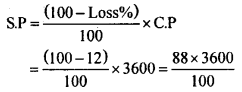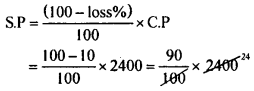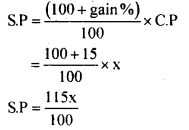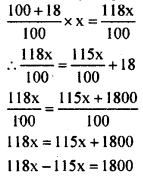# KSEEB Solutions for Class 8 Maths Chapter 9 Commercial Arithmetic Ex 9.2

In this chapter, we provide KSEEB SSLC Class 8 Maths Chapter 9 Commercial Arithmetic Ex 9.2 for English medium students, Which will very helpful for every student in their exams. Students can download the latest KSEEB SSLC Class 8 Maths Chapter 9 Commercial Arithmetic Ex 9.2 pdf, free KSEEB SSLC Class 8 Maths Chapter 9 Commercial Arithmetic Ex 9.2 pdf download. Now you will get step by step solution to each question.

### Karnataka State Syllabus Class 8 Maths Chapter 9 Commercial Arithmetic Ex 9.2

Question 1.
Sonu bought a bicycle for Rs. 3750 and spent Rs. 250 on its repairs. He sold it for Rs. 4400. Find his loss or profit percentage.
C.P = 3750 + 250 = Rs. 4000 S P = Rs. 4400
S.P > C.P
∴ S.P – C.P = 4400 – 4000 = Rs. 400
Protit percent =$\frac { profit }{ C.P }$ × 100$\frac { 400}{ 4000 }$ × 100 = 10
Profit percent = 10%

Question 2.
A shop keeper purchases an article for Rs. 3500 and pays the transport charge of Rs. 100. He incurred a loss of 12% in selling this. Find selling price of the article.
C.P = 3500 + 100 = Rs. 3600
Loss percent = 12%S.P = Rs.3168.

Question 3.
By selling a watch for Rs. 720, Ravi loses 10%. At what price should he sell it, in order to gain 15% .
S.P = Rs. 720,
loss percent = 10% C.P = ?
CP=100(100− Loss percent )×SP=100(100−10)×720=10090×720
C.P = RS. 800
gain percent = 15 %
S.P = ?
S.P=(100+gain%)100×C.P=100+15100×800=115100×800
S.P = Rs. 920
He should sell it for Rs. 920 to gain a 15% profit.

Question 4.
Hari bought two fans for Rs. 2400 each. He sold one at a loss of 10% and the other at a profit of 15%. Find the selling price of each fan and also the total profit or loss.
C.P = Rs. 2400, Loss% = 10%
S.P = ?S.P = Rs. 2160
∴ When the loss percent is 10% S.P. is Rs. 2160
C.P = Rs. 2400
profit percent = 15%
S.P = ?
S.P=(100+ Profit %)100×C.P=100+15100×2400=115100×2400
S.P. = Rs. 2760.
When the profit percent is 15%, the S.P. is Rs. 2760.
Total C.P. of two fans is 2400 + 2400 = Rs. 4800.
Total S.P. of two fans is 2160 + 2760 = Rs. 4920.
Total SP > Total C.P
∴ Total profit = Total S.P – Total C.P = 4920-4800 Profit = Rs. 120.

Question 5.
A storekeeper sells a book at a 15% gain. Had he sold it for Rs. 18 more. He would have gained 18%. Find the cost price of the book.
Let the C.P be Rs. x
gain % = 15%Had he sold it for Rs. 18 more the new S.P.
would be Rs. 115×100 . This is equal to S.P
When the gain percent is 18%
S.P when gain percent is 18% is118 x – 115 x = 1800
3 x = 1800
x = 18003
x = 600
∴ Cost price = Rs.600.

Question 6.
The cost price of 12 pens is equal to the selling price of 10 pens. Find the profit percentage.
Let the C.P of one pen be Rs. 1
∴ C.P of 12 pens = Rs. 12
Given S.P. of 10 pens = C.P. of 12 pens.
S.P. of 10 pens = Rs. 12 C.P of 10 pens = Rs. 10
∴ S.P > C.P So there is gain
Gain = S.P – C.P= 12 – 10 = 2
Gain percent = GainC.P × 100
= 210 × 100 = 20 %
Gain percent = 20%

All Chapter KSEEB Solutions For Class 8 maths

—————————————————————————–

All Subject KSEEB Solutions For Class 8

*************************************************

I think you got complete solutions for this chapter. If You have any queries regarding this chapter, please comment on the below section our subject teacher will answer you. We tried our best to give complete solutions so you got good marks in your exam.

If these solutions have helped you, you can also share kseebsolutionsfor.com to your friends.

Best of Luck!!